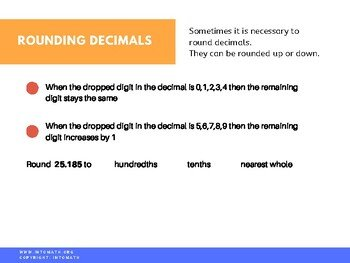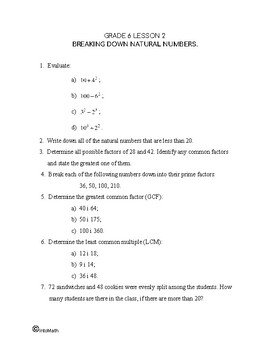CA\$2.00

You will practice how to determine the place value of the digits within the decimal and why it is important to understand the place value of each digit when converting decimals to percent.

For example, does 0.1 represent 10% or 1%? Why? You would need to multiply 0.1 by 100 to find the percent equivalent of this decimal, which results in 10%. It is easy to multiply any decimal by 100, since all you have to do is just move a decimal point 2 digits to the right of the original position.